A functor which is "injective on Hom-sets" . Explicitly, a functor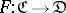is called faithful if, given any two morphisms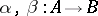in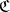with the same domain and codomain, the equation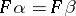implies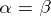. The name derives from the representation theory of groups: a permutation (respectively,-linear) representation of a groupis faithful if and only if it is faithful when considered as a functor(respectively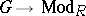). A faithful functor reflects monomorphisms (that is,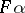monic impliesmonic) and epimorphisms; hence if the domain categoryis balanced (i.e. has the property that any morphism which is both monic and epic is an isomorphism) then it also reflects isomorphisms. A functor with the latter property is generally called conservative; however, some authors include this condition in the definition of faithfulness.